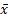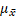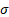It looks like you're using Internet Explorer 11 or older. This website works best with modern browsers such as the latest versions of Chrome, Firefox, Safari, and Edge. If you continue with this browser, you may see unexpected results.

# Math

Unless otherwise stated, the material in this guide is from The Learning Centre at Centennial College.  Content has been adapted for the NWP Learning Commons in March 2022. This work is licensed under a Creative Commons BY 4.0 International License.Normal Distribution

The Central Limit Theorem states that the averages (means) of randomly selected groups (samples) of data points taken from a population have an approximately normal distribution, whether the population is normally distributed or not. This is very useful: it means we can apply the normal distribution tools to find probabilities to almost any significantly large population!

A sampling distribution is a picture of what we get when we randomly select groups of data points from our population, and calculate the means of those groups (the sample means). So, instead of having all the data points from the population plotted for a picture, we plot the means of these samples.

The more data points you have in each sample, the more normal these means are distributed. The standard size for samples when using the Central Limit Theorem is $$n\geq 30$$ though smaller samples are permitted if the population is normally distributed to begin with.

As the sample size increases, the spread of the values decreases (the distribution gets ‘narrower’ when we draw a picture). A decrease in spread means a decrease in the variance and standard deviation, so the standard deviation of the sampling distribution changes accordingly:

$\sigma_{\bar{x}} = \frac{\sigma}{\sqrt{n}}$

This information is used to rewrite the Z formula for cases when, instead of using data from a whole population, we are using data from the distribution of the sample means:

$z=\frac{\bar{x} - \mu_\bar{x}}{\frac{\sigma}{\sqrt{n}}}$= sample mean= mean of the sampling distribution (= population mean)= standard deviation of the population

n = number of data points in each sample

This video provides a review of the definition of the Central Limit Theorem: